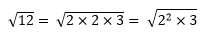Checkout JEE MAINS 2022 Question Paper Analysis : Checkout JEE MAINS 2022 Question Paper Analysis :

# Square Root Of 12

The square root of 12 is represented in the radical form as 12, which is equal to 2√3.  Since 2√3 cannot be further simplified, hence such roots are called surds. Apart from representing the value of root 12 in radical form, it can also be written in the decimal form, such as √12 = 3.464, up to three places of decimals. Now the question comes how to find the value of root 12? 12 is not a perfect square like numbers such as 2, 3, 5, 6, 24, 13, 125, etc. Hence, its simplification is a little typical. But numbers like 4, 16, 25, 81, 121, etc. are the perfect squares and its easy to estimate the square roots.  We get square of a number when we multiply the number by itself. Let us first take the examples of a few squares.

For example,

112  = 11 × 11 = 121

92 = 9 × 9 = 81

52 = 5 × 5 = 25

Therefore, in the above examples, 11, 9 and 5 are the square numbers. But if we have to find out that a number is a perfect square or not, we need to check the unit place of the number.

• If at the unit place, the number ends with 2, 3, 7 and 8, then the number is not a perfect square.
• A perfect square number ends with 1, 4, 5, 6 and 9.

The symbol represents the square root, ‘√’. This symbol ‘√’ is called a radical symbol or radix. The number underneath the radical symbol or radix is called as radicand.

## How to Find Value of Root 12?

The root of 12 is represented in the form of √12. Number 12 is an even number and not a prime number. Prime numbers have only two factors, 1 and the number itself, such as 1, 3, 5, etc. As we know, 12 have six factors, 1, 2, 3, 4, 6 and 12 itself, such as,

1 × 12 = 12

2 × 6 = 12

3 × 4 = 12

4 × 3 = 12

6 × 2 = 12

12 × 1 = 12

But the question comes, how can we find out the square root value of 12? First, let us write the factors of 12 as given below.

12 = 2 × 2 × 3

You can see, in the above expression, there is only one square number available on the right-hand side. Therefore, the value of the root of 12 can be written as;Taking the square term out of the root we get,

√12 = 2 √3

This is the radical form of √12. We can also write it in decimal form, by putting the value of √3 which is approximately 1.73. Hence,

√12 = 2 × 1.73

√12 = ±3.46 approximately.

Like 12, there are also many numbers which are not perfect squares. For example, 18, 20, 27, etc. are not perfect squares, as they give the value in radical form or decimal form.

Also, find:

## Video Lessons on Square Roots

### Visualising square roots### Finding Square roots### Table of Squares and Square Root From 1 to 15

To simplify questions based on the square root of numbers from 1 to 15, we are providing here the table of squares and square root, so that it is easy to identify which numbers are perfect squares and which are not.

 Number Squares Square Root (Upto 3 places of decimal) 1 12 = 1 √1 = 1 2 22 = 4 √2 = 1.414 3 32 = 9 √3 = 1.732 4 42 = 16 √4 = 2.000 5 52 = 25 √5 = 2.236 6 62 = 36 √6 = 2.449 7 72 = 49 √7 = 2.646 8 82 = 64 √8 = 2.828 9 92 = 81 √9 = 3.000 10 102 = 100 √10 = 3.162` 11 112 = 121 √11 = 3.317 12 122 = 144 √12 = 3.464 13 132 = 169 √13 = 3.606 14 142 = 196 √14 = 3.742 15 152 = 225 √15 = 3.873

Download BYJU’S – The Learning App and learn to find the squares and roots of numbers with the help of interactive videos.

Test your Knowledge on Square Root Of 12